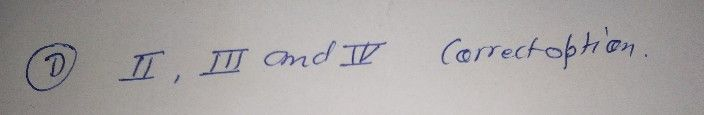Symbol
Problem$Pr0$ $A0$ $A8sossm0n$ $oin0aions$ Choose the letter of the best answer, Write the chosen $c+400$ on a separato $cncα$ of paper. $1$ Which of the following is true about the system of linear equntions in two variables? 1. Any set of linear equations. $11$ A set of two or more linear equations in two variables. $11$ $Asy$ system of linear equations may have only one solution, infinitely many solutions, or no solution at all. $1V.$ A system of linear equations in two variables can be solved algebraically $or$ graphically. A. I and 11 $C.1$ I, II, and III $B$ Il $an0$ III D. II, III, and $1V$ Which of the following equations could NOT be considered as system of linear equation in two variables? $A$ $\left(7x-y=8$
7th-9th grade
Other
Search count: 124
SolutionQanda teacher - Lokesh